# Connection object

(diff) ← Older revision | Latest revision (diff) | Newer revision → (diff)

A differential-geometric object on a smooth principal fibre bundlethat is used to define a horizontal distributionof a connection in. Let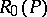be the bundle of all tangent frames tosuch that the firstvectors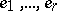are tangent to the corresponding fibre, and are generated bybasis elements in the Lie algebra of the structure groupof,. A connection object then consists of functions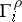onsuch that the subspace ofis spanned by the vectors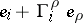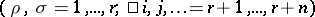. Furthermore, themust satisfy the following conditions on: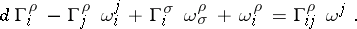(1)

They are expressed by using the-forms onthat occur in the structure equations for the forms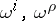given by the co-basis dual to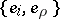;(2)

A connection object also defines a corresponding connection form, given by the relation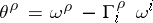, and its curvature form, given by the formulas: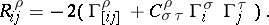For example, letbe the space of affine tangent frames of an-dimensional smooth manifold. Then the second equation in (2) has the formand (1) reduces to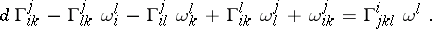Under parallel displacement one must have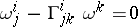. If a local chart is chosen in, and if in its domain one makes the transition to the natural frame of the chart, i.e.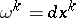, then the parallel displacement is defined by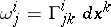. The classical definition of a connection object of an affine connection onis given by the set of functionsdefined on the domains of the charts such that under transition to the coordinates of another chart these functions are transformed according to the formulas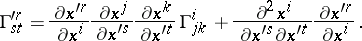Here this follows from the condition of invariance under displacement.

How to Cite This Entry:
Connection object. Encyclopedia of Mathematics. URL: http://encyclopediaofmath.org/index.php?title=Connection_object&oldid=13997
This article was adapted from an original article by Ãœ. Lumiste (originator), which appeared in Encyclopedia of Mathematics - ISBN 1402006098. See original article